# Support Vector Machine

$hθ(x)=g(θTx)g(z)=11+e−z$
• 如果要$y=1$，需要${h}_{\theta }\left(x\right)\approx 1$，即${\theta }^{T}x>>0$

• 如果要$y=0$，需要${h}_{\theta }\left(x\right)\approx 0$，即${\theta }^{T}x<<0$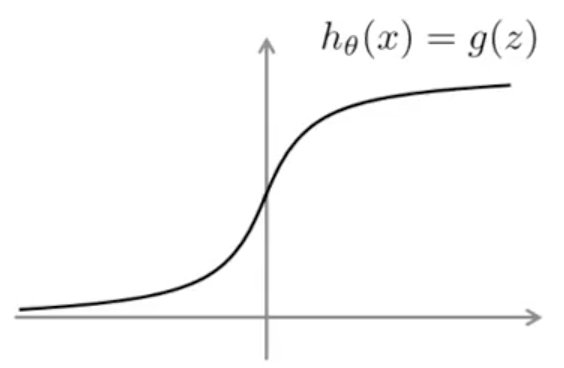$Cost of example : − y log ⁡ ( h θ ( x ) ) − ( 1 − y ) log ⁡ ( 1 − h θ ( x ) )$

$z={\theta }^{T}x$, 带入上面式子得到

$Cost of example : - y log ( 1 1+e−θTx ) - ( 1-y ) log ( 1 - 1 1+e−θTx )$
• $y=1$时，后一项为零，上述式子为：$-\mathrm{log}\left(\frac{1}{1+{e}^{-{\theta }^{T}x}}\right)$

• $y=0$时，后一项为零，上述式子为： $-\mathrm{log}\left(1-\frac{1}{1+{e}^{-{\theta }^{T}x}}\right)$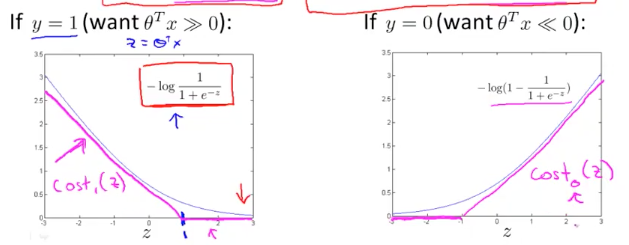$min θ 1 m [ ∑ i = 1 m y (i) ( - log ( h θ ( x ( i ) ) ) ) + ( 1 − y (i) ) ( ( - log ( 1 − h θ ( x ( i ) ) ) ) ] + λ 2 m ∑ j = 1 n θ j 2$

${\mathrm{cost}}_{1}\left(z\right)$${\mathrm{cost}}_{0}\left(z\right)$ 替换中括号中的第一项和第二项，得到：

$A+λB-> CA+B$

SVM 的 Cost 函数为:

SVM 的预测函数为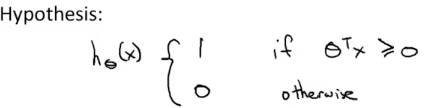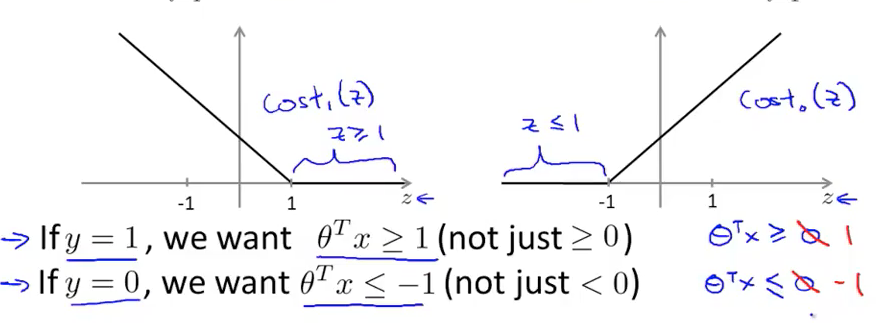• 如果要$y=1$，我们希望${\theta }^{T}x>=1$，此时${\mathrm{cost}}_{1}\left(z\right)$的值为 0

• 如果要$y=0$，我们希望${\theta }^{T}x<=-1$，此时${\mathrm{cost}}_{0}\left(z\right)$的值为 0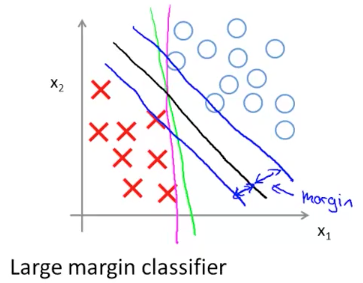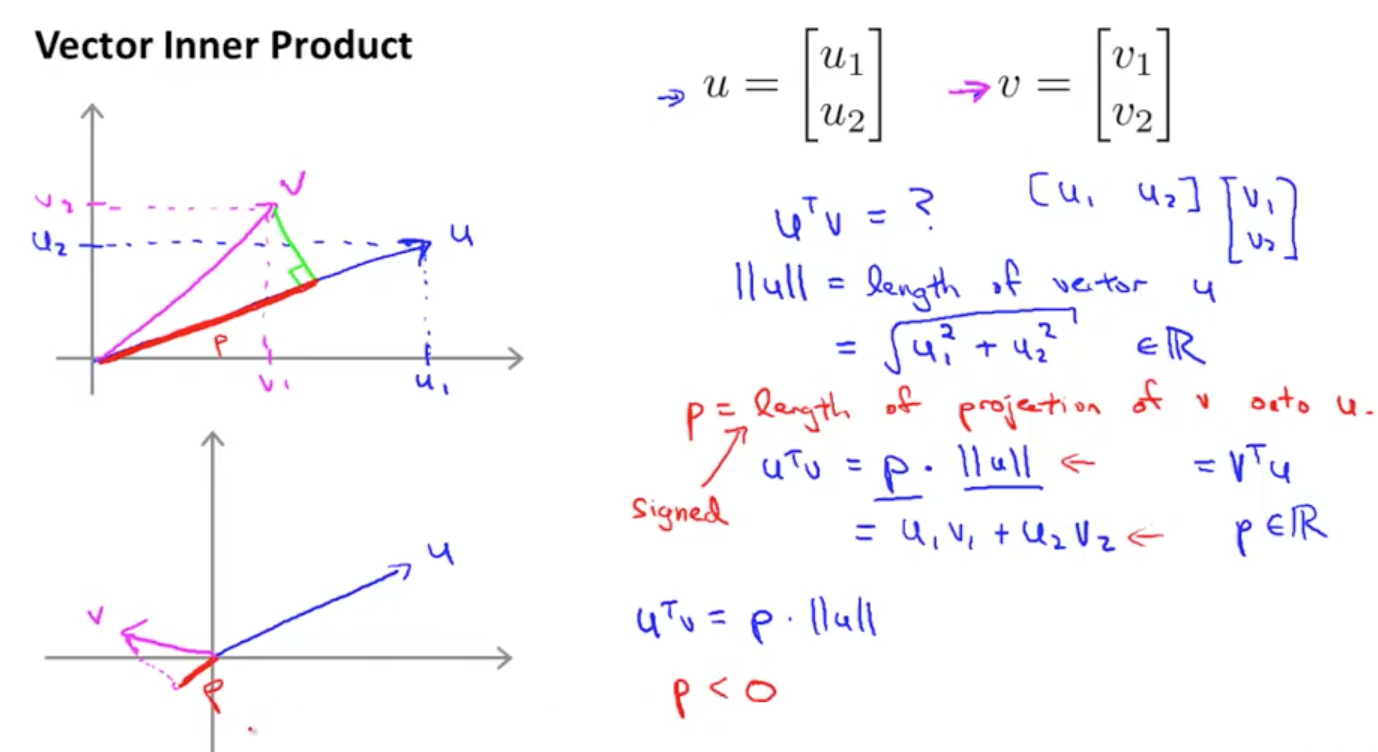$uTv = p||u||$

SVM 代价函数的优化目标为：

$min θ 12 ∑ j = 1 n θ j 2$ $θTx(i) >= 1 if y(i) = 1$ $θTx(i) <= -1 if y(i) = 0$

$min θ 12 ∑ j = 1 n θ j 2 = 12 ( θ12 + θ22 ) = 12 || θ ||2$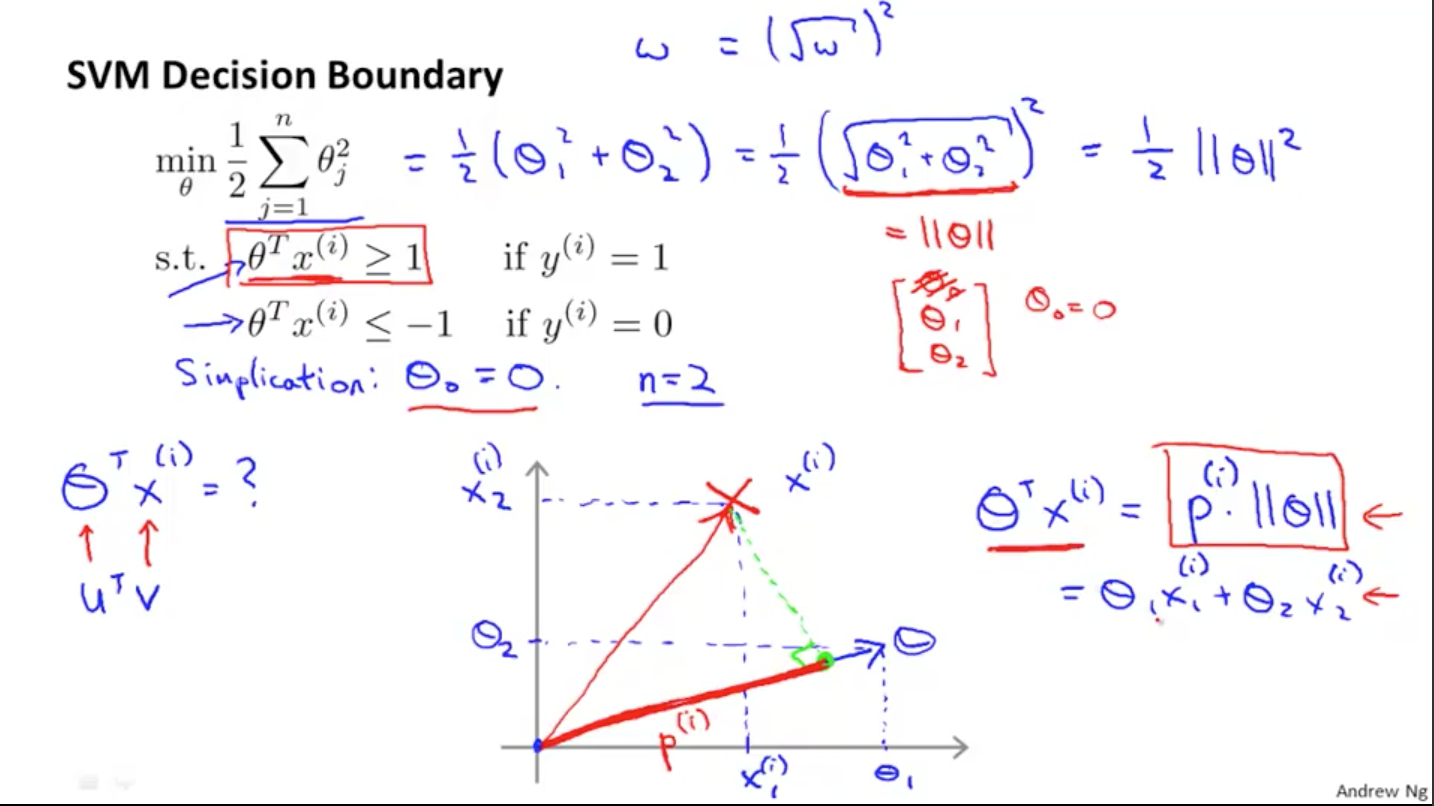$θTx(i) = p(i) ||θ|| = θ1x1(i) + θ2x2(i)$

$min θ 12 ∑ j = 1 n θ j 2$ $p(i) ||θ|| >= 1 if y(i) = 1$ $p(i) ||θ|| <= -1 if y(i) = 0$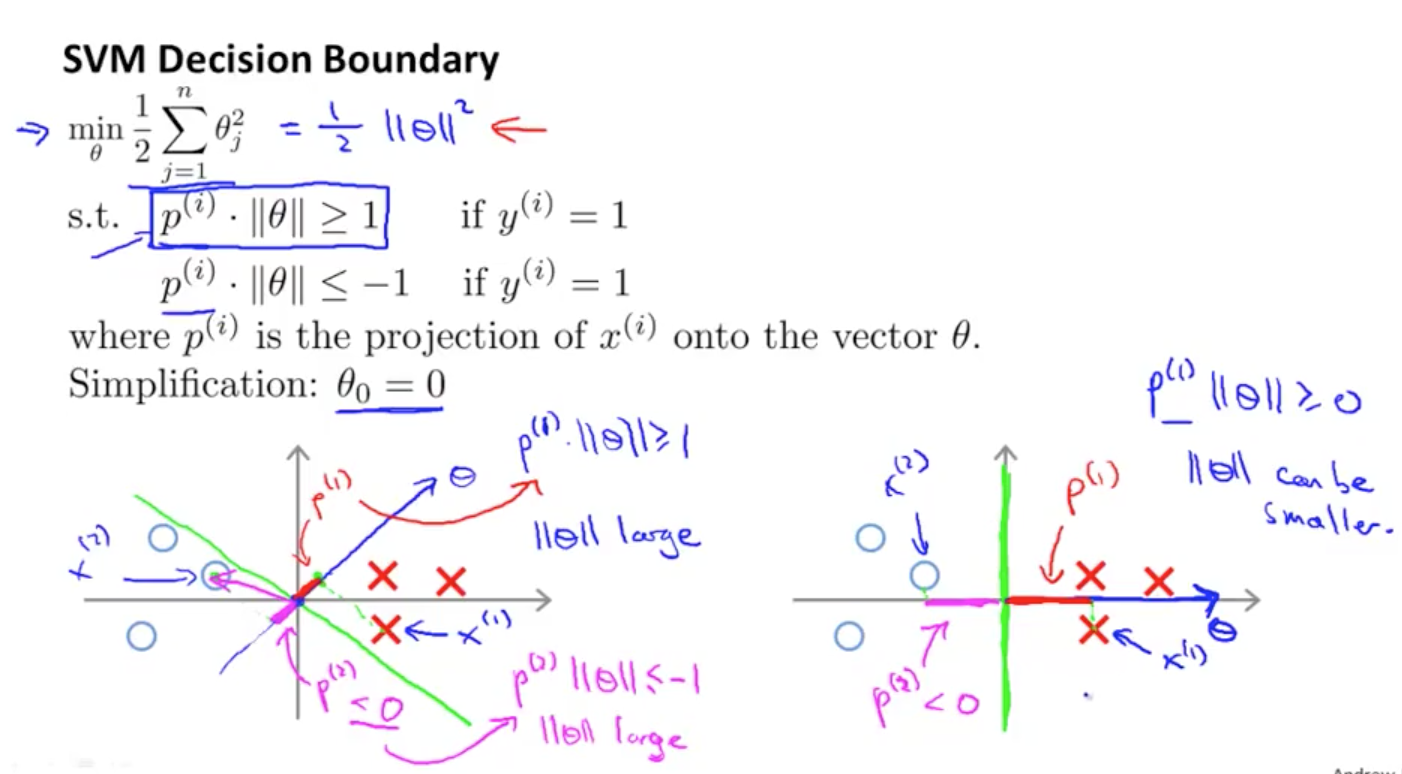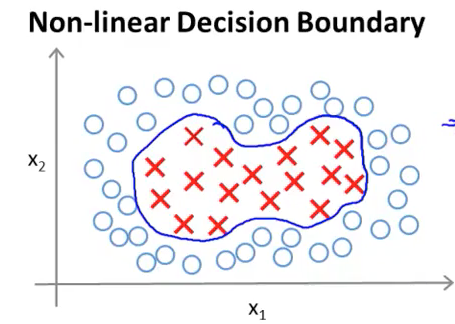$h (θ) = { 1 if θ0 + θ1x1 + θ2x2 + θ3x1x2 + θ4x12 + θ5x22 + ...>=0 0 otherwise$

$f$替换多项式中的$x$，有

$θ0 + θ1f1 + θ2f2 + θ3f3+...$

$f1=x1 f2=x2 f3=x1x2 f4=...$

$fi = similarity ( x , l(i) ) = exp ( - ||x- l(i) ||2 2 σ2 ) = exp ( - ∑ j = 1 n ( xj - lj(i) )2 2 σ2 )$

• ${l}^{\mathrm{\left(i\right)}}$

成为 Landmark ，每个标记点会定义一个新的 feature 变量，选取方式在后面将会介绍

• similarity 函数称为 Kernel 函数，Kernel 函数有多种，在上述公式中，kernel 函数为高斯函数，有时也记作：$K\left(x,{l}^{\mathrm{\left(i\right)}}\right)$
• 假设$x\approx {l}^{\mathrm{\left(i\right)}}$，则有${f}_{i}\approx \text{exp}\left(-\frac{0}{2{\sigma }^{2}}\right)\approx 1$
• 假设$x$和 landmark，即${l}^{\mathrm{\left(i\right)}}$很远，则有${f}_{i}\approx \text{exp}\left(-\frac{\text{(large number)}}{2{\sigma }^{2}}\right)\approx 0$
• 给定一个新的样本$x$，我们可以计算${f}_{1},{f}_{2},{f}_{3}$的值

• 高斯核函数 Octave 实现
function sim = gaussianKernel(x1, x2, sigma)
%RBFKERNEL returns a radial basis function kernel between x1 and x2
%   sim = gaussianKernel(x1, x2) returns a gaussian kernel between x1 and x2
%   and returns the value in sim
x1 = x1(:); x2 = x2(:);

sim = e^(-(sum((x1-x2).^2)/(2*sigma^2)));

% =============================================================

end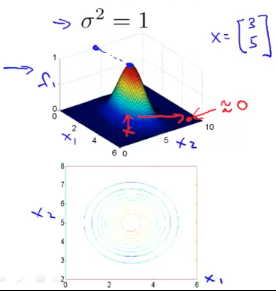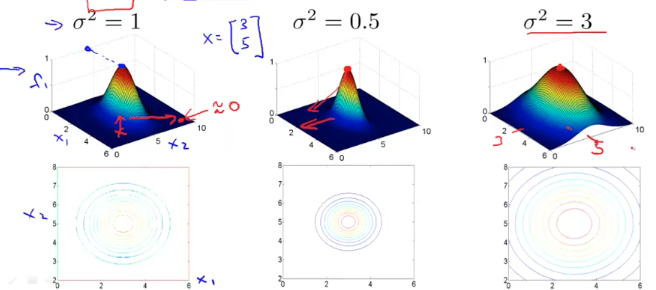• 上图可以看到当${\sigma }^{2}$越小时，f 下降到 0 的速度越快，反之则越慢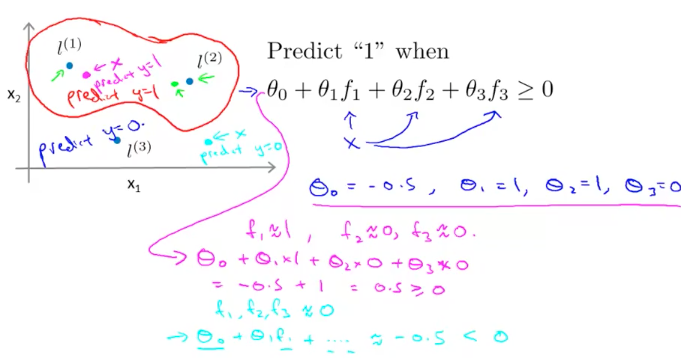$θ0 + θ1 * 1 + θ2 * 0 + θ3 * 0 = -0.5+1 = 0.5 >= 0$

$θ0 + θ1 * 0 + θ2 * 0 + θ3 * 0 = -0.5+0 = -0.5 < 0$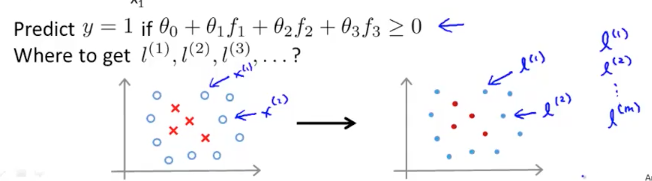$f = [ f0 =1 f1 = similarity ( x , l(1) ) f2 = similarity ( x , l(2) ) ... fm = similarity ( x , l(m) ) ]$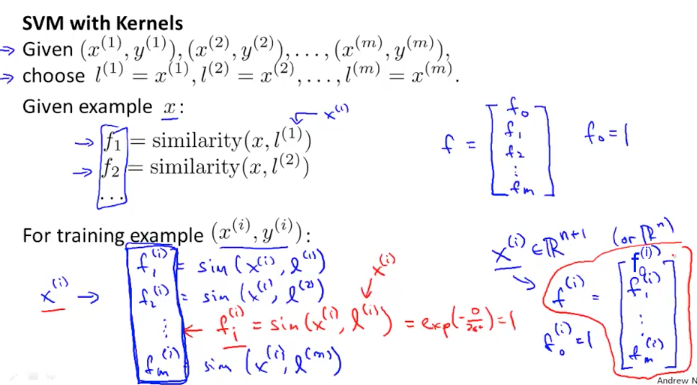$h(θ) = { 1 if θTf>=0 0 otherwise$

• 某些 SVM 算法，在 Regularization 一项会有不同的表示方式，有的将$\frac{1}{2}\sum _{j=1}^{m}{\theta }_{j}^{2}$ 表示为 ${\theta }^{T}\theta$，数学上是等价的。也有些算法为了计算效率将其表示为${\theta }^{T}M\theta$$M$为样本数量，暂不关心其中的细节。对于最优化函数的解法，这个课程不讨论，调用现有的函数即可。

• SVM 参数 - 选择$C\left(=\frac{1}{\lambda }\right)$的值。$C$值很大，会造成较低偏差（bias），但会带来较高的方差(variance)，有 overfit 的趋势；$C$值很小，会造成较高的偏差和较低的方差，有 underfit 的趋势。

	- 选择$<msup><mi>σ</mi><mn>2</mn></msup>$的值，$<msup><mi>σ</mi><mn>2</mn></msup>$越大，$<mi>f</mi>$下降的越平滑，会导致样本点$<msup><mi>x</mi><mi>(i)</mi></msup>$据landmark点$<msup><mi>l</mi><mi>(i)</mi></msup>$的距离偏大，从而带来较大的偏差和较低的方差，有underfit的趋势。$<msup><mi>σ</mi><mn>2</mn></msup>$越小，$<mi>f</mi>$下降速度越快，越陡峭，会带来较低的偏差和较高的方差，有overfit的趋势。

• 参数选择的 Octave 实现思路

function [C, sigma] = dataset3Params(X, y, Xval, yval)
%DATASET3PARAMS returns your choice of C and sigma for Part 3 of the exercise
%where you select the optimal (C, sigma) learning parameters to use for SVM
%with RBF kernel
%   [C, sigma] = DATASET3PARAMS(X, y, Xval, yval) returns your choice of C and
%   sigma. You should complete this function to return the optimal C and
%   sigma based on a cross-validation set.
%

% You need to return the following variables correctly.
C = 1;
sigma = 0.3;

% ====================== YOUR CODE HERE ======================
% Instructions: Fill in this function to return the optimal C and sigma
%               learning parameters found using the cross validation set.
%               You can use svmPredict to predict the labels on the cross
%               validation set. For example,
%                   predictions = svmPredict(model, Xval);
%               will return the predictions on the cross validation set.
%
%  Note: You can compute the prediction error using
%        mean(double(predictions ~= yval))
%

values = [ 0.01, 0.03, 0,1, 0.3, 1, 3, 10, 30 ];

max_error = Inf;

for c = values;
for s = values;
model       = svmTrain(X, y, c, @(x1, x2) gaussianKernel(x1, x2, s));
predictions = svmPredict(model, Xval);
error = mean(double(predictions ~= yval));
if error < max_error;
max_error = error;
C=c;
sigma=s;
end;
end
end;

% =========================================================================

end


### SVM 编程实现思路

• 给出参数$C$的值
• 给出 Kernel 的核函数 - 如果样本特征$n$很大，而样本数量$m$很少的的情况下使用，可以选择”No kernel”，所谓”No kernel”实际上就是”Linear kernel”，使用线性方程 ${\theta }^{T}x={\theta }_{0}+{\theta }_{1}{x}_{1}+{\theta }_{2}{x}_{2}+{\theta }_{3}{x}_{3}+\cdots +{\theta }_{n}{x}_{n}$ 来判定$y$值 - 如果样本特征$n$很少，而样本数量$m$很大，可以选择高斯 kernel，使用高斯 kernel 要注意几点 - 给出合适的${\sigma }^{2}$的值。 - 对样本进行 feature scaling，不同 feature 间的数量级可能不同，避免范数计算产生较大偏差，对 feature 要进行归一化 - 如果要使用其它的核函数，要确保该核函数满足”Mercer’s Theorem”，这样 SVM 库才能对核函数进行通用的最优化求解 - 对于多个核函数，选择在交叉验证数据集表现最好的核函数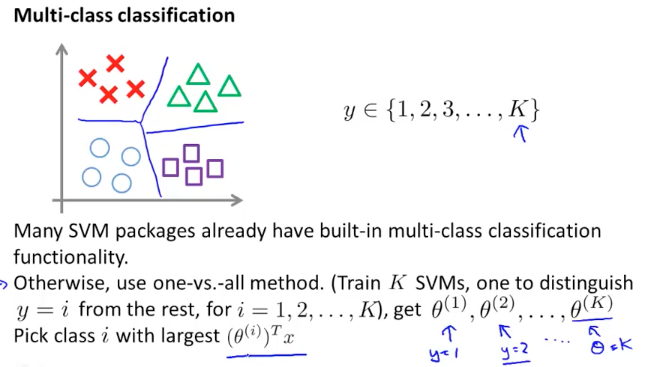### Logistic regression vs. SVM

• 如果 $n>m$(n=10,000,m=10~1000)，比如 Spam 邮件过滤，有几千个关键词作为 feature，而邮件数量即样本数量要明显少于特征数量，这时可以使用逻辑回归或者 SVM 线性核函数
• 如果 $n$很小(1 ~ 1000)，$m$处于中等数量级（10 ~ 10000），这时可以使用 SVM 高斯核函数
• 如果 $n$很小(1 ~ 1000)，$m$很大（几十万），这种情况下使用 SVM 高斯核函数会很慢，这时需要增加更多的 feature，然后使用逻辑回归或者线性 SVM
• 对于神经网络来说没有这些限制，但是训练起来比较慢